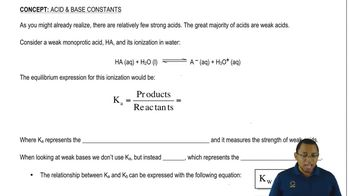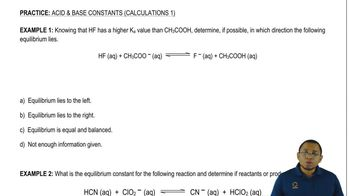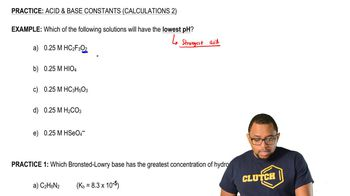Start typing, then use the up and down arrows to select an option from the list.Jules Bruno
200views
3
Hey, guys, In this new video, we're gonna put to practice some of the concepts you learned about K and K B. So the first example says, knowing that HF has a larger or higher K value than ch three C O h, which is acetic acid. Determine if possible, in which direction the following equilibrium lies. All right. So we learned earlier that the higher your K value, the stronger you are as an acid. So HF has, ah higher K value. So it's a stronger acid than acetic acid is. So we're gonna say H f is the stronger acid. And again it's stronger because it has a higher K value and it's the stronger acid, so the acetic acid on the product side would be the weaker acid. Now we have to say on which side is the equilibrium lie. All you have to realize here is weaker means more stable in chemistry. Weaker just means less energy. Less energy means you're more stable, and the equilibrium will always lie on the side with the weaker acid. Because the weaker acid is the more stable acid, so are weaker. Acid is on the right side here, so we're gonna say that equilibrium lies to the right. It's a simple is that first you have to know that higher K means stronger acid and then just figure out which side the weaker acid would be on. Wherever the weaker ass it is, that's where your equilibrium will lie.01:2003:5101:3407:0901:53